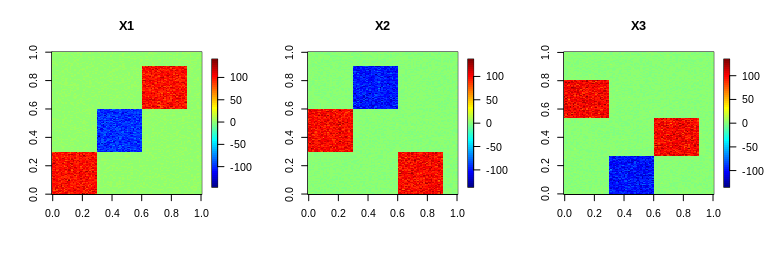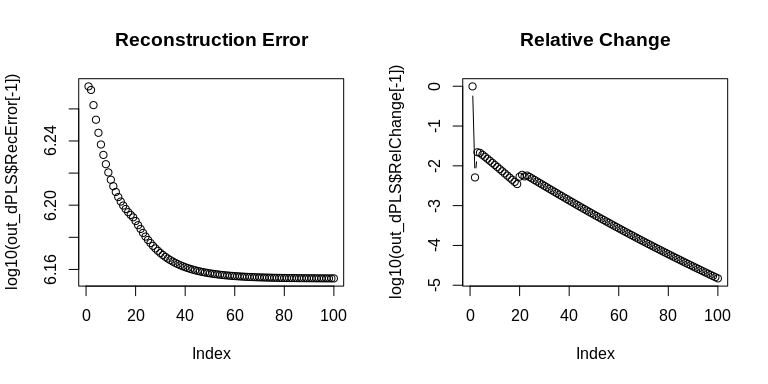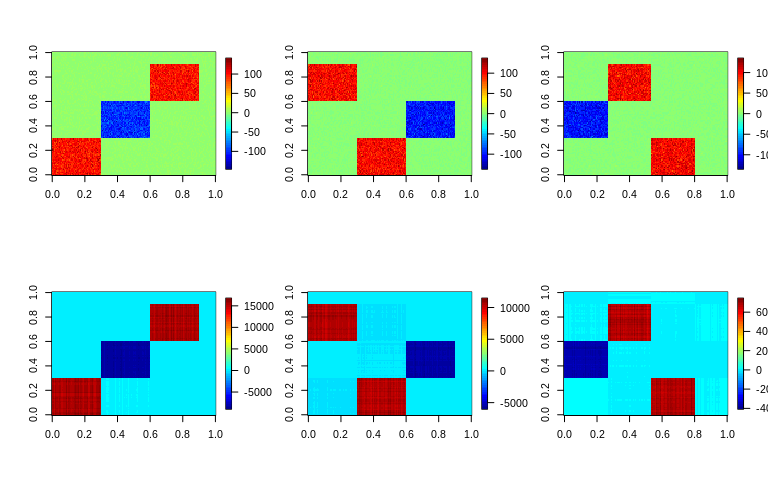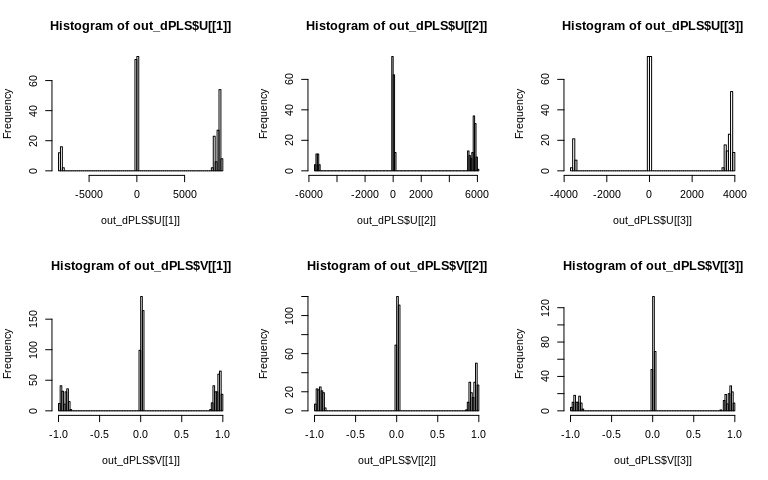# 5. Discretized Partial Least Squares (dPLS)

Laboratory for Bioinformatics Research, RIKEN Center for Biosystems Dynamics Research

# Introduction

In this vignette, we consider approximating multiple matrices as a product of ternary (or non-negative) low-rank matrices (a.k.a., factor matrices).

Test data is available from toyModel.

library("dcTensor")
X <- dcTensor::toyModel("dPLS_Easy")

You will see that there are five blocks in the data matrix as follows.

suppressMessages(library("fields"))
layout(t(1:3))
image.plot(X[], main="X1", legend.mar=8)
image.plot(X[], main="X2", legend.mar=8)
image.plot(X[], main="X3", legend.mar=8)# Semi-Ternary Simultaneous Matrix Factorization (STSMF)

Here, we introduce the ternary regularization to take {-1,0,1} values in $$V_{k}$$ as below:

$\max{\mathrm{tr} \left( V_{j}'X_{j}'X_{k}V_{k} \right)}\ \mathrm{s.t.}\ j ≠k, V \in \{-1,0,1\},$ where $$j$$ and $$k$$ range from $$1$$ to $$K$$, $$K$$ is the number of matrices, $$X_{k}$$ ($$N \times M_{k}$$) is a $$k$$-th data matrix and $$V_{k}$$ ($$M_{k} \times J$$) is a $$k$$-th ternary loading matrix. In dcTensor package, the object function is optimized by combining gradient-descent algorithm (Tsuyuzaki 2020) and ternary regularization.

## Basic Usage

In STSMF, a rank parameter $$J$$ ($$\leq \min(N, M)$$) is needed to be set in advance. Other settings such as the number of iterations (num.iter) are also available. For the details of arguments of dPLS, see ?dPLS. After the calculation, various objects are returned by dPLS. STSMF is achieved by specifying the ternary regularization parameter as a large value like the below:

set.seed(123456)
out_dPLS <- dPLS(X, Ter_V=1E+5, J=3)
str(out_dPLS, 2)
## List of 6
##  $U :List of 3 ## ..$ : num [1:100, 1:3] 8722 8926 8821 8626 8589 ...
##   ..$: num [1:100, 1:3] 5888 5898 6044 5910 5695 ... ## ..$ : num [1:100, 1:3] 3879 3904 3961 3806 3909 ...
##  $V :List of 3 ## ..$ : num [1:300, 1:3] 0.96 0.966 0.973 0.95 0.925 ...
##   ..$: num [1:200, 1:3] 0.887 0.892 0.881 0.913 0.913 ... ## ..$ : num [1:150, 1:3] 0.0252 0.0332 0.0286 0.0346 0.0244 ...
##  $RecError : Named num [1:101] 1.00e-09 1.88e+06 1.87e+06 1.83e+06 1.79e+06 ... ## ..- attr(*, "names")= chr [1:101] "offset" "1" "2" "3" ... ##$ TrainRecError: Named num [1:101] 1.00e-09 1.88e+06 1.87e+06 1.83e+06 1.79e+06 ...
##   ..- attr(*, "names")= chr [1:101] "offset" "1" "2" "3" ...
##  $TestRecError : Named num [1:101] 1e-09 0e+00 0e+00 0e+00 0e+00 0e+00 0e+00 0e+00 0e+00 0e+00 ... ## ..- attr(*, "names")= chr [1:101] "offset" "1" "2" "3" ... ##$ RelChange    : Named num [1:101] 1.00e-09 9.92e-01 5.11e-03 2.20e-02 2.12e-02 ...
##   ..- attr(*, "names")= chr [1:101] "offset" "1" "2" "3" ...

The reconstruction error (RecError) and relative error (RelChange, the amount of change from the reconstruction error in the previous step) can be used to diagnose whether the calculation is converged or not.

layout(t(1:2))
plot(log10(out_dPLS$RecError[-1]), type="b", main="Reconstruction Error") plot(log10(out_dPLS$RelChange[-1]), type="b", main="Relative Change")The products of $$U_{k}$$ and $$V_{k}$$ ($$k = 1 \ldots K$$) show whether the original data matrices are well-recovered by dPLS.

recX <- lapply(seq_along(X), function(x){
out_dPLS$U[[x]] %*% t(out_dPLS$V[[x]])
})
layout(rbind(1:3, 4:6))
image.plot(t(X[]))
image.plot(t(X[]))
image.plot(t(X[]))
image.plot(t(recX[]))
image.plot(t(recX[]))
image.plot(t(recX[]))The histograms of $$V_{k}$$s show that all the factor matrices $$V_{k}$$ looks ternary.

layout(rbind(1:3, 4:6))
hist(out_dPLS$U[], breaks=100) hist(out_dPLS$U[], breaks=100)
hist(out_dPLS$U[], breaks=100) hist(out_dPLS$V[], breaks=100)
hist(out_dPLS$V[], breaks=100) hist(out_dPLS$V[], breaks=100)# Session Information

## R version 3.6.3 (2020-02-29)
## Platform: x86_64-conda-linux-gnu (64-bit)
## Running under: CentOS Linux 7 (Core)
##
## Matrix products: default
## BLAS/LAPACK: /home/koki/miniconda3/lib/libopenblasp-r0.3.17.so
##
## locale:
##   LC_CTYPE=en_US.UTF-8       LC_NUMERIC=C
##   LC_TIME=en_US.UTF-8        LC_COLLATE=en_US.UTF-8
##   LC_MONETARY=en_US.UTF-8    LC_MESSAGES=en_US.UTF-8
##   LC_PAPER=en_US.UTF-8       LC_NAME=C
##  LC_MEASUREMENT=en_US.UTF-8 LC_IDENTIFICATION=C
##
## attached base packages:
##  stats     graphics  grDevices utils     datasets  methods   base
##
## other attached packages:
##  nnTensor_1.1.12   fields_13.3       viridis_0.6.2     viridisLite_0.4.0
##  spam_2.8-0        dcTensor_1.0.1
##
## loaded via a namespace (and not attached):
##   Rcpp_1.0.8         highr_0.9          RColorBrewer_1.1-2 rTensor_1.4.8
##   bslib_0.3.1        compiler_3.6.3     pillar_1.7.0       jquerylib_0.1.4
##   tools_3.6.3        dotCall64_1.0-1    digest_0.6.29      jsonlite_1.8.0
##  evaluate_0.15      lifecycle_1.0.1    tibble_3.1.2       gtable_0.3.0
##  pkgconfig_2.0.3    rlang_0.4.11       DBI_1.1.2          yaml_2.3.5
##  xfun_0.29          fastmap_1.1.0      gridExtra_2.3      stringr_1.4.0
##  dplyr_1.0.6        knitr_1.37         generics_0.1.2     sass_0.4.0
##  vctrs_0.3.8        maps_3.4.0         plot3D_1.4         tidyselect_1.1.1
##  grid_3.6.3         glue_1.4.2         R6_2.5.1           fansi_1.0.2
##  tcltk_3.6.3        rmarkdown_2.11     purrr_0.3.4        ggplot2_3.3.5
##  magrittr_2.0.2     scales_1.1.1       htmltools_0.5.2    ellipsis_0.3.2
##  MASS_7.3-55        tagcloud_0.6       misc3d_0.9-1       assertthat_0.2.1
##  colorspace_2.0-3   utf8_1.2.2         stringi_1.7.6      munsell_0.5.0
##  crayon_1.5.0